Sri Yantra Intersections

 Marma Points The triple intersection points (where three lines meet) in the Sri Yantra are often correlated to the marma points on the human body. They can be subdivided in two general groups according to the following criteria:

GROUP 1: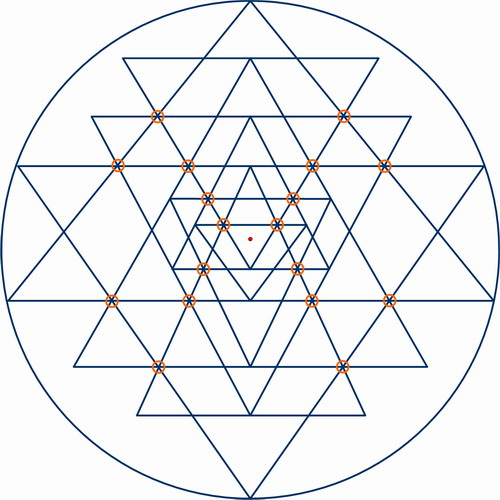The first group includes the points that result from three line crossing. In this configuration there is 18 such intersections.

GROUP 2: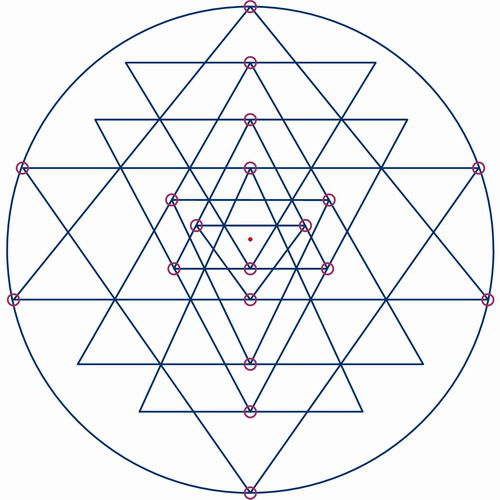The second group is includes the points that result from the apex of a triangle touching the base of another triangle or the outer circle. In this configuration there is 19 such intersections. Four more points are possible if triangle D5 and U4 touch the outer circle. We can further subdivide this group into 3 subgroups.

GROUP 2A: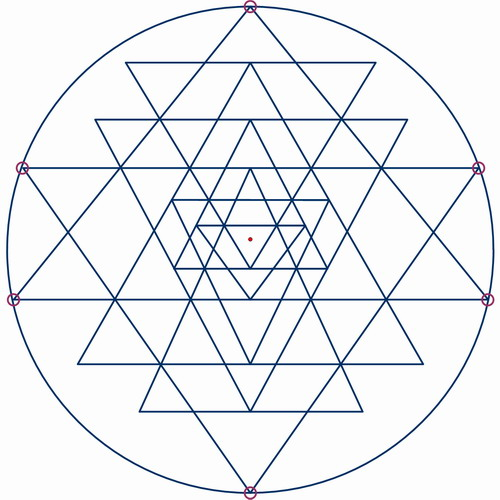There a 6 points where the apex of a triangle touches the outside circle. In some configurations this number can go up to 10.

GROUP 2B: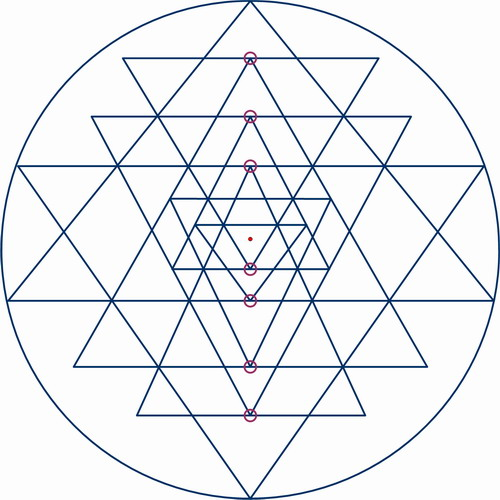7 points where the apex of a triangle touches the base of another triangle.

GROUP 2C: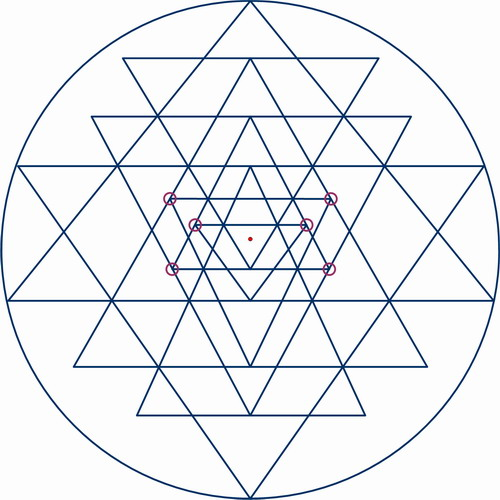6 points where the apex of a triangle touches the side of another triangle.